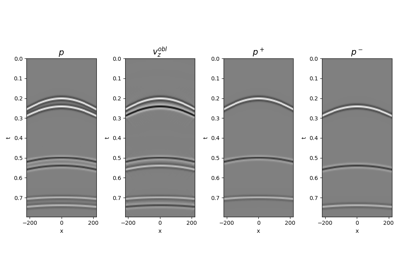# pylops.waveeqprocessing.UpDownComposition2D#

pylops.waveeqprocessing.UpDownComposition2D(nt, nr, dt, dr, rho, vel, nffts=(None, None), critical=100.0, ntaper=10, scaling=1.0, backend='numpy', dtype='complex128', name='U')[source]#

2D Up-down wavefield composition.

Apply multi-component seismic wavefield composition from its up- and down-going constituents. The input model required by the operator should be created by flattening the separated wavefields of size $$\lbrack n_r \times n_t \rbrack$$ concatenated along the spatial axis.

Similarly, the data is also a flattened concatenation of pressure and vertical particle velocity wavefields.

Parameters
ntint

Number of samples along the time axis

nrint

Number of samples along the receiver axis

dtfloat

Sampling along the time axis

drfloat

rhofloat

Density $$\rho$$ along the receiver array (must be constant)

velfloat

Velocity $$c$$ along the receiver array (must be constant)

nfftstuple, optional

Number of samples along the wavenumber and frequency axes

criticalfloat, optional

Percentage of angles to retain in obliquity factor. For example, if critical=100 only angles below the critical angle $$|k_x| < \frac{f(k_x)}{c}$$ will be retained will be retained

ntaperfloat, optional

Number of samples of taper applied to obliquity factor around critical angle

scalingfloat, optional

Scaling to apply to the operator (see Notes for more details)

backendstr, optional

Backend used for creation of obliquity factor operator (numpy or cupy)

dtypestr, optional

Type of elements in input array.

namestr, optional

New in version 2.0.0.

Name of operator (to be used by pylops.utils.describe.describe)

Returns
UDoppylops.LinearOperator

Up-down wavefield composition operator

UpDownComposition3D

3D Wavefield composition

WavefieldDecomposition

Wavefield decomposition

Notes

Multi-component seismic data $$p(x, t)$$ and $$v_z(x, t)$$ can be synthesized in the frequency-wavenumber domain as the superposition of the up- and downgoing constituents of the pressure wavefield ($$p^-(x, t)$$ and $$p^+(x, t)$$) as follows :

$\begin{split}\begin{bmatrix} \hat{p} \\ \hat{v_z} \end{bmatrix}(k_x, \omega) = \begin{bmatrix} 1 & 1 \\ \frac{k_z}{\omega \rho} & - \frac{k_z}{\omega \rho} \\ \end{bmatrix} \begin{bmatrix} \hat{p^+} \\ \hat{p^-} \end{bmatrix}(k_x, \omega)\end{split}$

where the vertical wavenumber $$k_z$$ is defined as $$k_z=\sqrt{\frac{\omega^2}{c^2} - k_x^2}$$.

We can write the entire composition process in a compact matrix-vector notation as follows:

$\begin{split}\begin{bmatrix} \mathbf{p} \\ s\mathbf{v_z} \end{bmatrix} = \begin{bmatrix} \mathbf{F} & 0 \\ 0 & s\mathbf{F} \end{bmatrix} \begin{bmatrix} \mathbf{I} & \mathbf{I} \\ \mathbf{W}^+ & \mathbf{W}^- \end{bmatrix} \begin{bmatrix} \mathbf{F}^H & 0 \\ 0 & \mathbf{F}^H \end{bmatrix} \mathbf{p^{\pm}}\end{split}$

where $$\mathbf{F}$$ is the 2-dimensional FFT (pylops.signalprocessing.FFT2), $$\mathbf{W}^\pm$$ are weighting matrices which contain the scalings $$\pm \frac{k_z}{\omega \rho}$$ implemented via pylops.basicprocessing.Diagonal, and $$s$$ is a scaling factor that is applied to both the particle velocity data and to the operator has shown above. Such a scaling is required to balance out the different dynamic range of pressure and particle velocity when solving the wavefield separation problem as an inverse problem.

As the operator is effectively obtained by chaining basic PyLops operators the adjoint is automatically implemented for this operator.

1

Wapenaar, K. “Reciprocity properties of one-way propagators”, Geophysics, vol. 63, pp. 1795-1798. 1998.

## Examples using pylops.waveeqprocessing.UpDownComposition2D#14. Seismic wavefield decomposition

14. Seismic wavefield decomposition